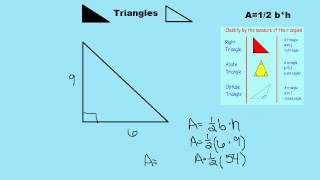## Ashley JaafarAshley Jaafar Teacher United States of America Education is my passion.

## Work and education

Bachelor degree
Rutgers University

## User videosArea of Triangles
This lesson will go through examples and explain how to find the area of triangles. The formula of area will be explained. This lesson will look at example problems of how to find the area of triangles. The lesson will give an explanation of how to use the are formula and will take a step by step look to better explain the concept.Order of Operations
This lesson will look at an example problem on order of operations. An explanation of how to use the order of operations to solve a problem along with an explanation of PEMDAS. This lesson will take a step by step look to better explain the concept.